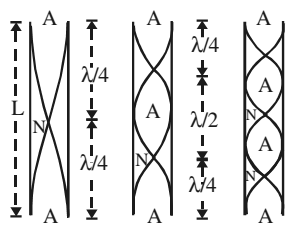# Organ Pipes

It is the simplest form of a wind instrument. A wooden or metal pipe producing musical sound is known as organ pipe. Flute is an example of organ pipe.

If both the ends of the pipe are open, it is an open pipe. However, if one end is closed, it is a closed pipe. When you blow in gently, almost a pure tone is heard. This pure tone is called a fundamental note. But, when you blow hard, you can also hear notes of frequencies which are integral multiple of the frequency of the fundamental note.

At the closed end of a pipe, there can be no motion of the air particles and the closed end must be node.

At the open end of the pipe, the change in density must be zero since this end is in communication with atmosphere. Since the strain is zero, hence this end must be an antinode.

### Open Pipe

The simplest mode of vibrations of the air column is called fundamental mode. At each end, there is an antinode and between two antinodes, there is a node.Since the distance between a node and next antinode is λ/4, the length l of the pipe is

l = (λ/4) + (λ/4) = λ/2

λ = 2l

The frequency of the note produced is

n1 = v/λ = v/2l

In the next mode of vibration of the air column, one more node and one more antinode has been produced. In this case

λ = (λ/4) + (λ/4) + (λ/4) + (λ/4) = l

The frequency of the note is

n2 = v/λ = v/l = 2v/2l

n2 = 2n1

The note produced is called second harmonic or 1st overtone. To get the second harmonic, you have to blow harder. But if you blow still harder one more node and one more antinode is produced. In this case

λ = 2l/3

Therefore, the frequency of the note emitted is

n3 = 3n1

The note produced is called the 3rd harmonic or 2nd overtone.

### Closed Pipe

There is an antinode at the open end and a node at the closed end.The wave length of the wave produced is given by

l = λ/4

λ = 4l

The frequency of the note emitted is

n1 = v/λ = v/4l

The note produced is called fundamental note. On blowing harder one more node and antinode will be produced. The wavelength of the note produced is given by

l = λ/2 + λ/4 = 3λ/4

λ = 4l/3

The frequency of the note emitted is

n3 = v/λ = 3v/4l = 3n1

The note produced is called the first overtone or the 3rd harmonic of the fundamental. Blowing still harder one more node and one more antinode will be produced. The wavelength of the note produced is then given by

l = 5λ/4

λ = 4l/5

The frequency of the note emitted then will be

n5 = 5n1

The note produced is called the second overtone or the 5th harmonic of the fundamental.

On comparison with the notes emitted by the open and closed pipe, the open pipe is richer in overtones. In closed pipe, the even order harmonics are missing.